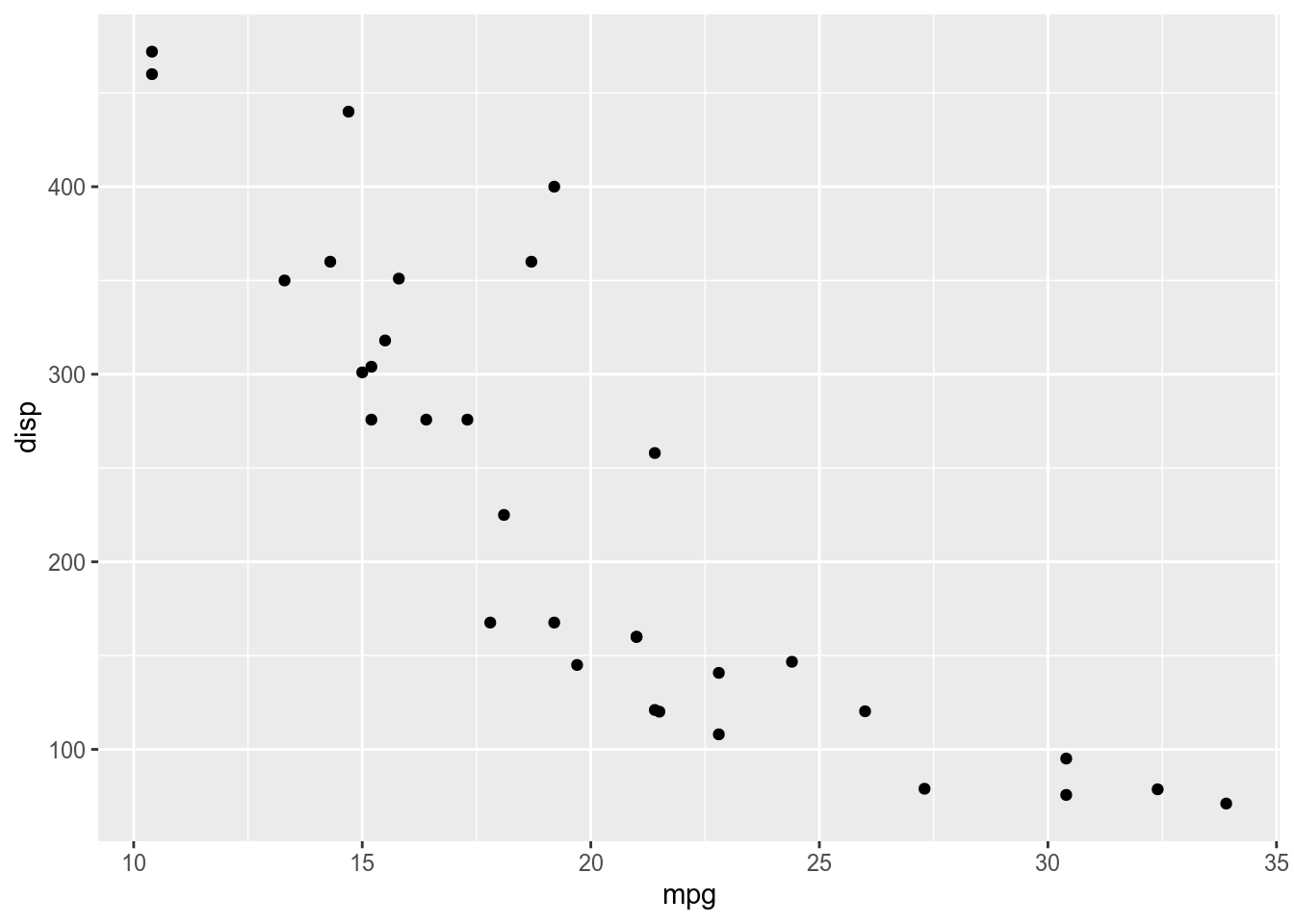## Installation

Get the stable version from CRAN:

install.packages("checkdown")

… or get the development version from GitHub:

install.packages("devtools")
devtools::install_github("agricolamz/checkdown")

## 1. Demo

The main goal of this package to create checking fields and boxes in rmarkdown. It could be used in class, when teacher share materials and tasks (as an .html page or an .html slides), so student can solve some problems and check themselves. It is really important since some students are too shy to ask a question, so you can create tasks that will check on the fly the understanding of the class material and give some hints to those students that get stuck. In contrast to the learnr package the checkdown package works without shiny and could be stored as a simple .html page (e. g. on Github Pages). In contrast to the exams output the checkdown package creates intaractive autochecking tasks. The interactive virsion of the exams output is bind to Blackboard Learn, that is really nice, but looks like an overkill for the simple task that checkdown solves.

library(checkdown)

### 1.1 Ask question with the check_question() function

Imagine that we want to create a checkbox with the answer 4. All you need is to create a following chunk with the chunck atribute results='asis' in your rmarkdown document:

check_question(answer =  4)

It is possible to change wrong and right answer’s messages using wrong and right arguments of the check_question() function. Let’s create some more questions.

Solve 3+3:

check_question(answer =  6, right = "correct", wrong = "not correct")

Type la-la:

check_question(answer =  "la-la")

Number of answers is not limited:

check_question(answer =  1:5)

It is also possible to create a list of answers for students to choose:

check_question("banana", options = c("apple", "banana", "bread"))

check_question("banana", options = c("apple", "banana", "bread"), type = "radio")

check_question(c("banana", "apple"), options = c("apple", "banana", "bread"), type = "checkbox")

If the list of possible answers is small, it is possible to align them in one line using alignment argument:

check_question("banana", options = c("apple", "banana", "bread"), type = "radio", alignment = TRUE)

You can shuffle answers using the random_answer_order argument:

check_question("banana", options = c("apple", "banana", "bread"), type = "radio", random_answer_order = TRUE)

If you don’t want to give the possibility of automatically check your question, just put NULL in the answer argument:

check_question(NULL, options = c("apple", "banana", "bread"), type = "radio")

### 1.2 Give some hints with the check_hint() function

Sometimes you know in advance what kind of mistakes will your students do. Some students are shy and don’t like asking questions, so hints could partially solve this problem. Again all you need is to create a following chunk with the chunck atribute results='asis' in your rmarkdown document:

check_hint("You can use the rmarkdown package")

Of course it is possible to change the message:

check_hint("You can use the rmarkdown package inside checkdown",
hint_title = "CLICK HERE")

It is possible to use Markdown inside messages:

check_hint("* You can use markdown **inside** the [chcekdown package](https://agricolamz.github.io/checkdown/)",
hint_title = "Click heRe")

Click heRe

check_question(answer =  4,
wrong = "a**R**e you su**R**e",
right = "### Right")

There is also a function for multiple hints:

check_hints(hint_text = c("look into the hint 2", "look into the hint 1"),
hint_title = c("hint 1", "hint 2"),
list_title = "list of hints")

list of hints

## 2. Inserting images

Sometimes it is nice to use images as a question. It also could be useful to insert images in hints. In order to do it you need to use insert_images() function, and enumerate the correct answer.

check_question(answer = 3,
options = insert_images(c("windows.png",
"mac.png",
"linux.png"),
image_width = 30))

Arguments image_width and image_height also except vector of values in case you need different size for different pictures. It is also possible to create a picture during the session, save it and use in checkdown functions.

tmp <- tempfile(fileext = ".png")
library(ggplot2)
ggplot(data = mtcars, aes(mpg, disp)) + geom_point()ggsave(filename = tmp)

Let’s use obtained picture in the hint (notice, how I used percantage in the image_width argument):

check_hint(paste("Here is our plot: <br>",
insert_images(tmp, image_width = "40%")))

• It is possible to avoid code in the output .html. Just use the chunk argument echo=FALSE.
• For now the package checkdown works only with html output and will not print anything for other otputs.
• Be careful with number precision: rounding could be different on different computers so if you ask your student to calculate log(3/4) it is possible that they will see only 6 or 7 numbers after the comma. So it make sence explicitly specify precision using round() function.
log(3/4)
check_question(answer =  round(log(3/4), 6))